### Home > MC2 > Chapter 5 > Lesson 5.3.3 > Problem5-112

5-112.
1. Simplify each numerical expression. Homework Help ✎

1.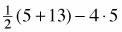2. (5 + 11) – (24 – 15) · (3)

3. 62 + 3 · 7 – 9 ÷ 3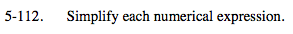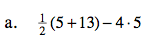First simplify the terms in parentheses, then exponents, the terms that are multiplied or divided, and finally the terms that are added or subtracted.

−11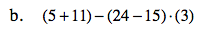See part (a).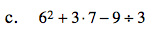See part (a).

54